# Function Composition CS 5010 Program Design Paradigms Bootcamp

• Slides: 29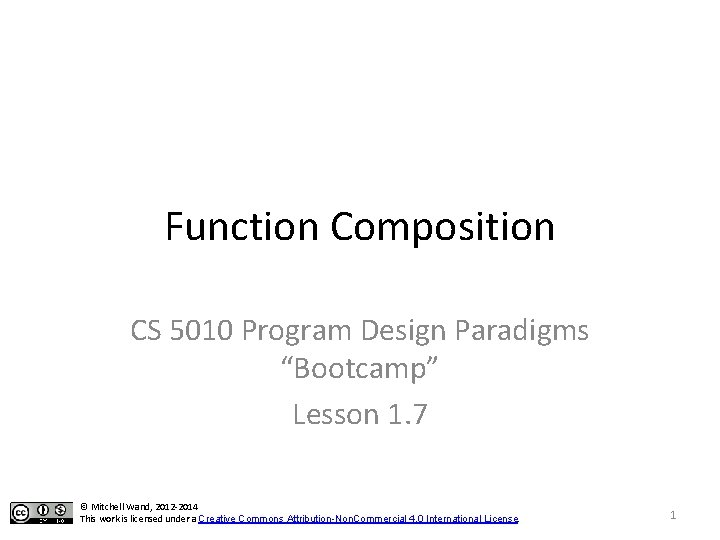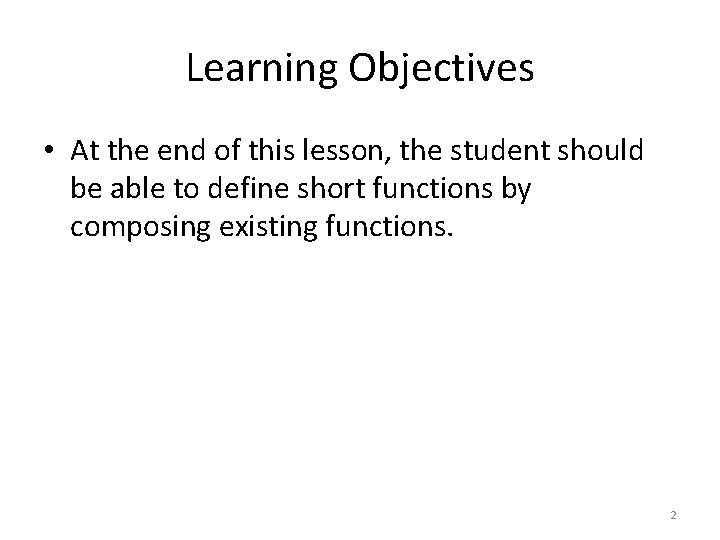Learning Objectives • At the end of this lesson, the student should be able to define short functions by composing existing functions. 2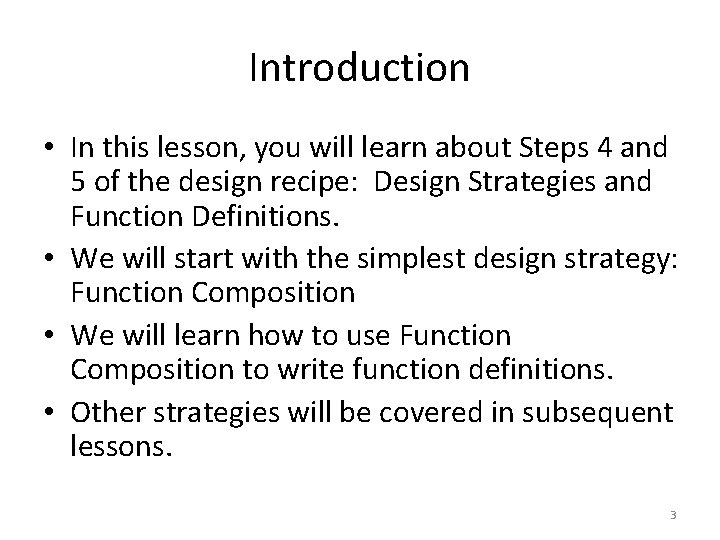Introduction • In this lesson, you will learn about Steps 4 and 5 of the design recipe: Design Strategies and Function Definitions. • We will start with the simplest design strategy: Function Composition • We will learn how to use Function Composition to write function definitions. • Other strategies will be covered in subsequent lessons. 3Programs are sets of Functions • We organize our programs as sets of functions. • A function takes an argument (or arguments) and returns a result. • The contract says what kind of data the argument and result are. • Purpose statement describes how the result depends on the argument. • The design strategy is a short description of how to get from the purpose statement to the code. 4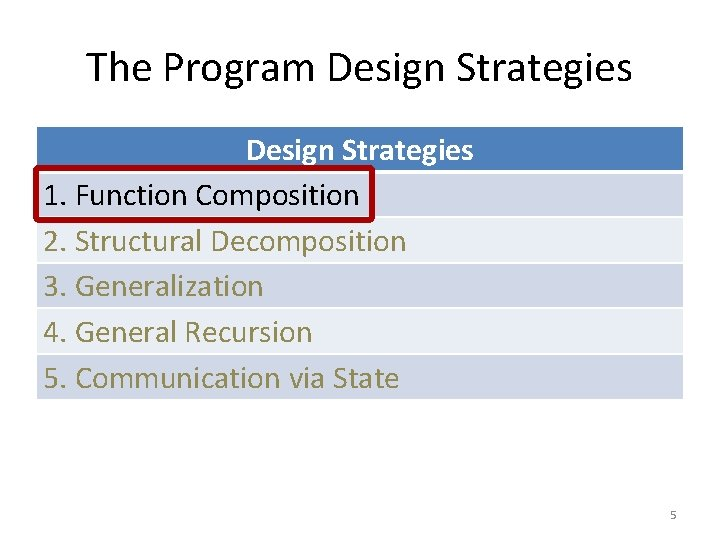The Program Design Strategies 1. Function Composition 2. Structural Decomposition 3. Generalization 4. General Recursion 5. Communication via State 5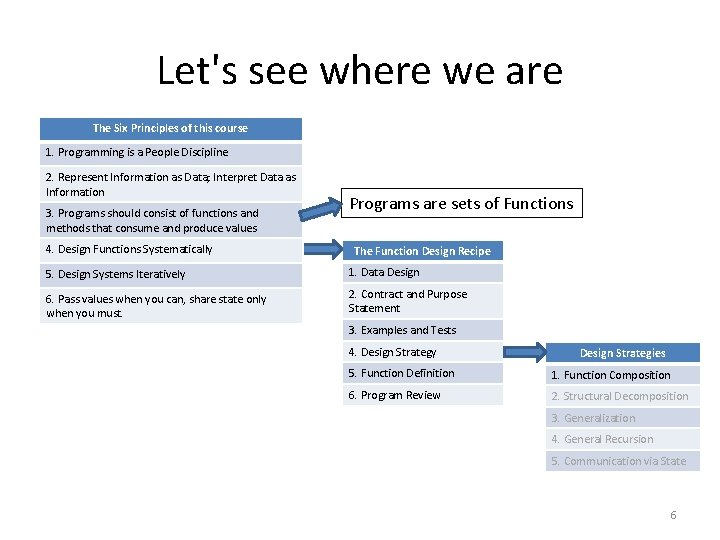Let's see where we are The Six Principles of this course 1. Programming is a People Discipline 2. Represent Information as Data; Interpret Data as Information 3. Programs should consist of functions and methods that consume and produce values 4. Design Functions Systematically Programs are sets of Functions The Function Design Recipe 5. Design Systems Iteratively 1. Data Design 6. Pass values when you can, share state only when you must. 2. Contract and Purpose Statement 3. Examples and Tests 4. Design Strategy Design Strategies 5. Function Definition 1. Function Composition 6. Program Review 2. Structural Decomposition 3. Generalization 4. General Recursion 5. Communication via State 6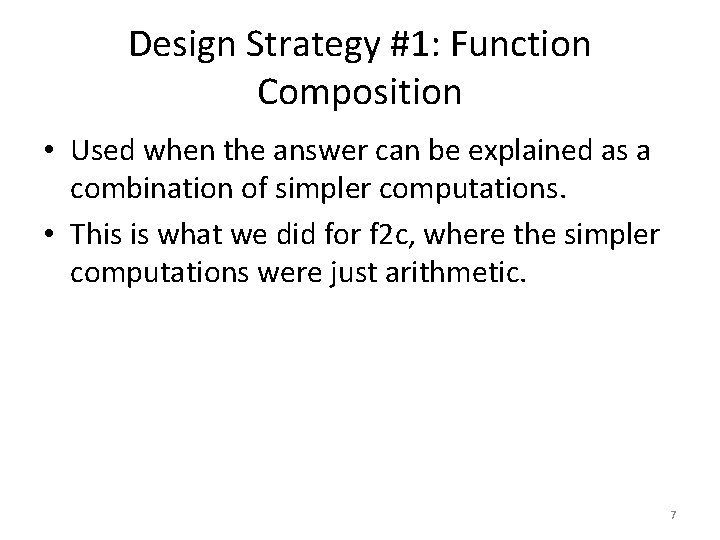Design Strategy #1: Function Composition • Used when the answer can be explained as a combination of simpler computations. • This is what we did for f 2 c, where the simpler computations were just arithmetic. 7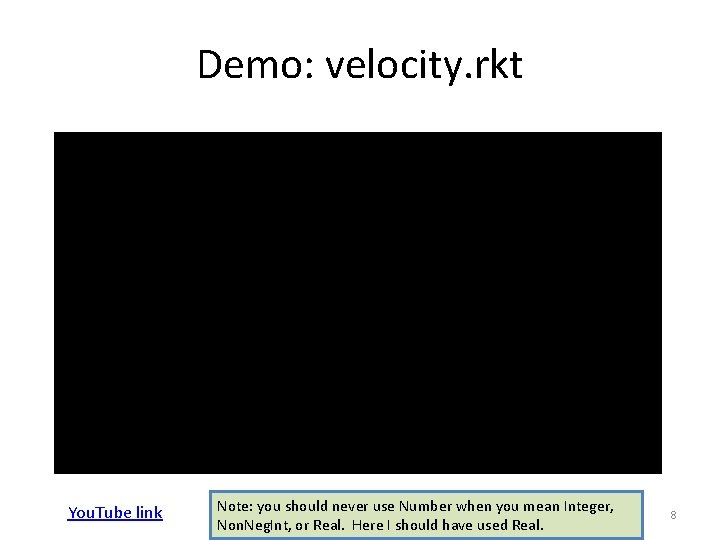Demo: velocity. rkt You. Tube link Note: you should never use Number when you mean Integer, Non. Neg. Int, or Real. Here I should have used Real. 8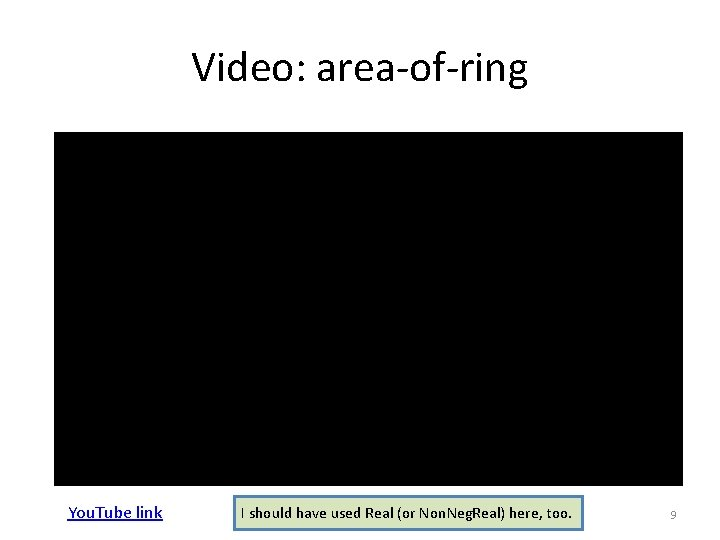Video: area-of-ring You. Tube link I should have used Real (or Non. Neg. Real) here, too. 9Function Compositions as Diagrams • We can think of a function composition as a wiring diagram. The arguments to the function flow in, and then they are steered (piped) through some functions. • In the next few slides, we’ll see some of the forms that this can take. • For each example, we’ll show a wiring diagram and the corresponding Racket expression. 10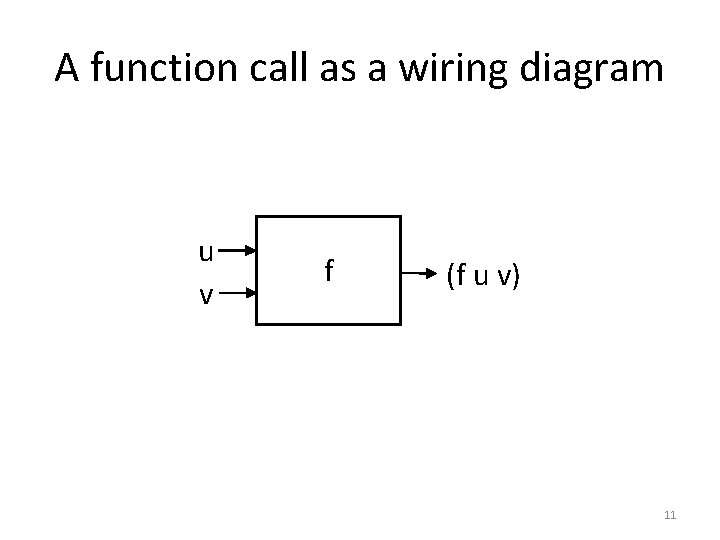A function call as a wiring diagram u v f (f u v) 11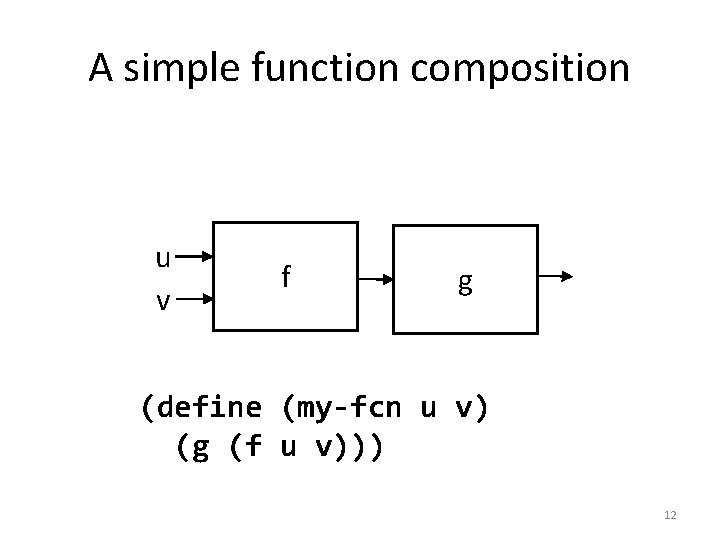A simple function composition u v f g (define (my-fcn u v) (g (f u v))) 12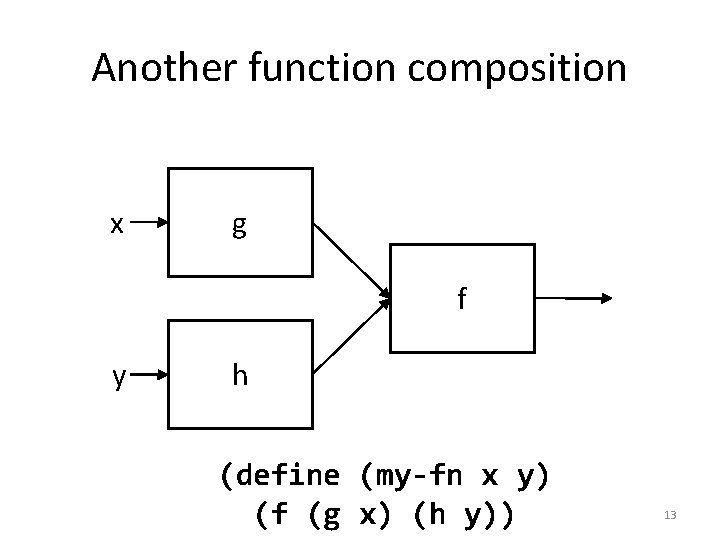Another function composition x g f y h (define (my-fn x y) (f (g x) (h y)) 13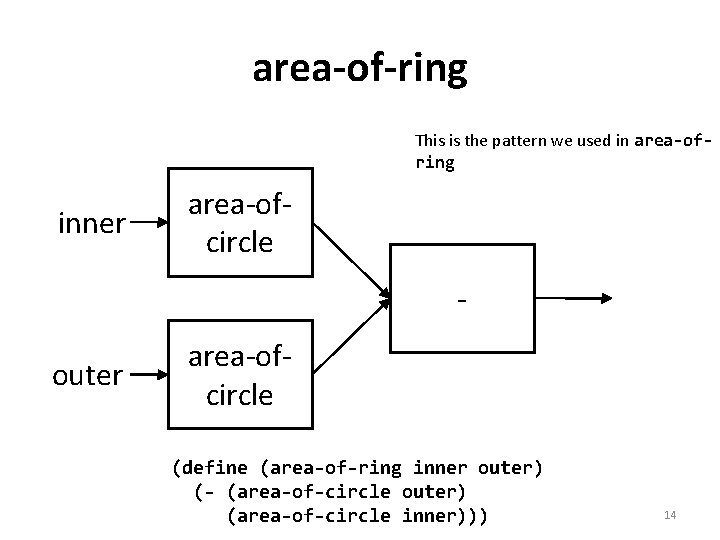area-of-ring This is the pattern we used in area-ofring inner area-ofcircle - outer area-ofcircle (define (area-of-ring inner outer) (- (area-of-circle outer) (area-of-circle inner))) 14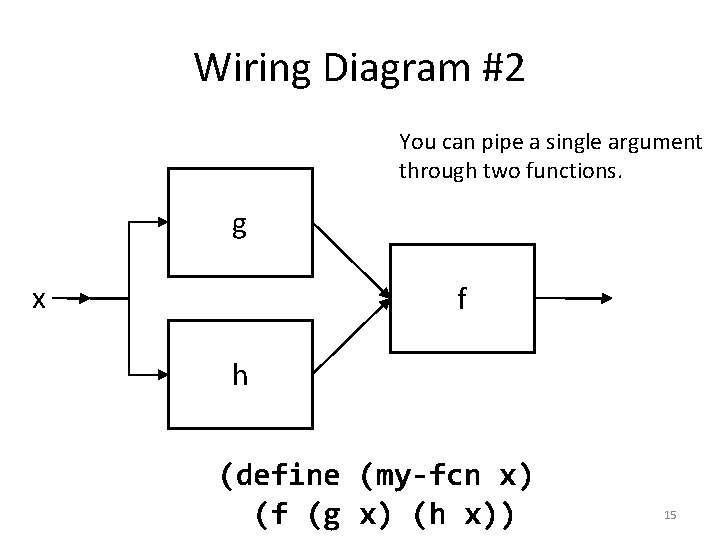Wiring Diagram #2 You can pipe a single argument through two functions. g x f h (define (my-fcn x) (f (g x) (h x)) 15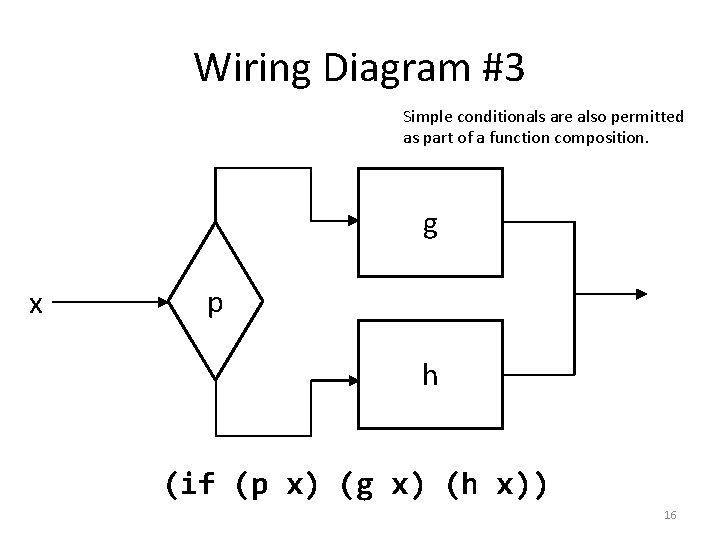Wiring Diagram #3 Simple conditionals are also permitted as part of a function composition. g x p h (if (p x) (g x) (h x)) 16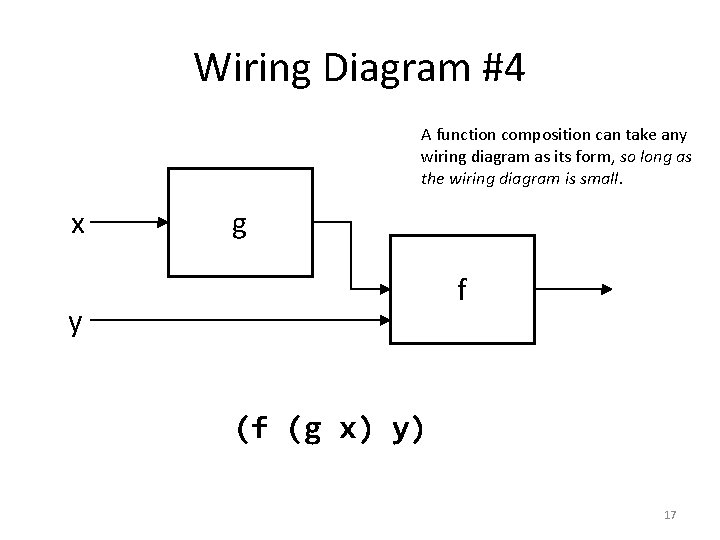Wiring Diagram #4 A function composition can take any wiring diagram as its form, so long as the wiring diagram is small. x g f y (f (g x) y) 17Definition of a function composition fc : : = variable : : = (function fc fc. . . ) : : = (if (pred var. . . ) fc But: no conditionals here. fc) Later on, we'll allow more complicated things in place of variables 18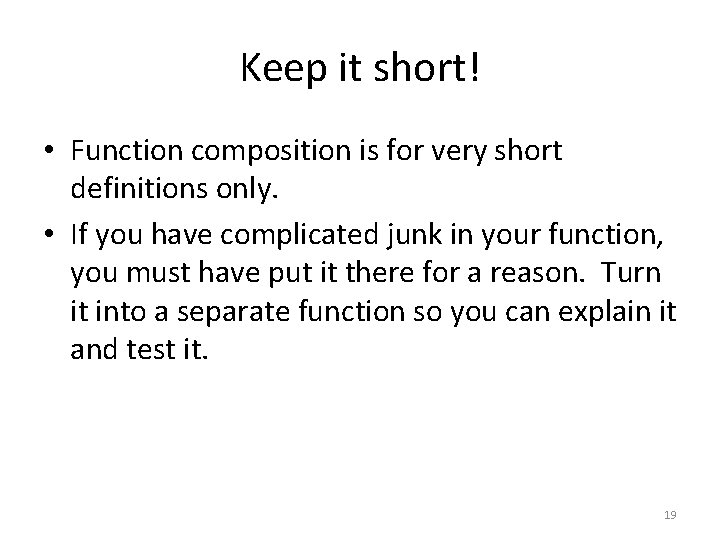Keep it short! • Function composition is for very short definitions only. • If you have complicated junk in your function, you must have put it there for a reason. Turn it into a separate function so you can explain it and test it. 19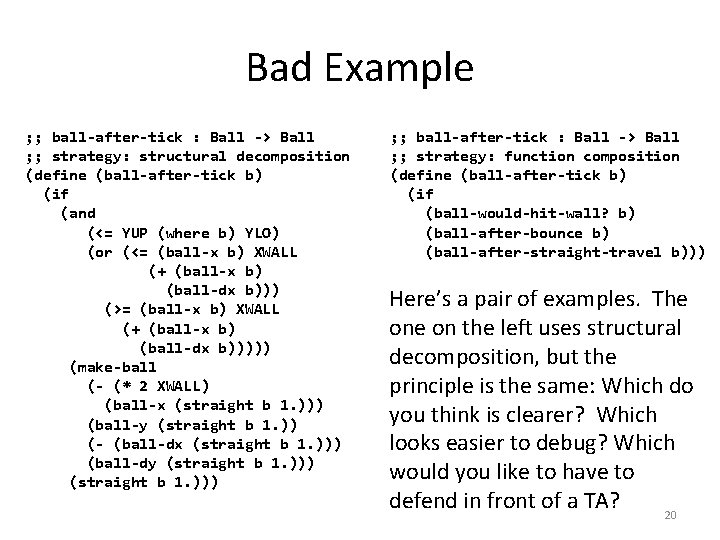Bad Example ; ; ball-after-tick : Ball -> Ball ; ; strategy: structural decomposition (define (ball-after-tick b) (if (and (<= YUP (where b) YLO) (or (<= (ball-x b) XWALL (+ (ball-x b) (ball-dx b))) (>= (ball-x b) XWALL (+ (ball-x b) (ball-dx b))))) (make-ball (- (* 2 XWALL) (ball-x (straight b 1. ))) (ball-y (straight b 1. )) (- (ball-dx (straight b 1. ))) (ball-dy (straight b 1. ))) ; ; ball-after-tick : Ball -> Ball ; ; strategy: function composition (define (ball-after-tick b) (if (ball-would-hit-wall? b) (ball-after-bounce b) (ball-after-straight-travel b))) Here’s a pair of examples. The on the left uses structural decomposition, but the principle is the same: Which do you think is clearer? Which looks easier to debug? Which would you like to have to defend in front of a TA? 20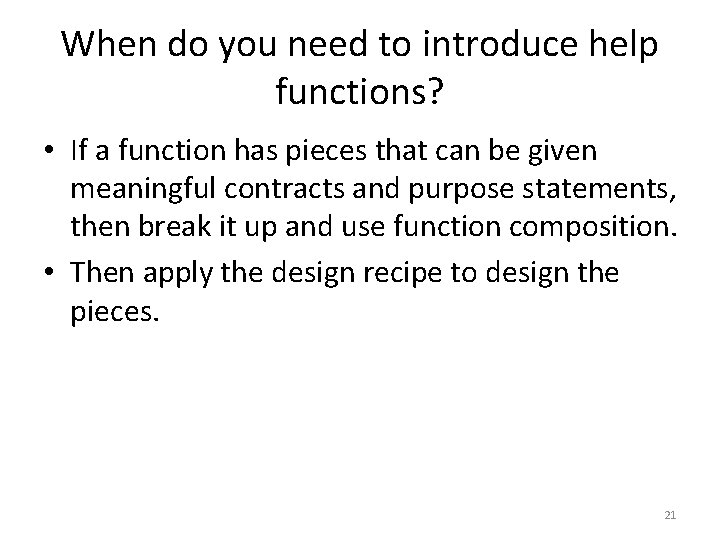When do you need to introduce help functions? • If a function has pieces that can be given meaningful contracts and purpose statements, then break it up and use function composition. • Then apply the design recipe to design the pieces. 21Cases: a small extension to Function Composition • Sometimes you need to break up a scalar data type into cases. • Do this with a cond. • This looks like a template, but it's dealing with scalar data, not itemized data. • This is one of the "special-purpose" strategies we mentioned in Lesson 1. 1, Slide 19. 22Example: ; ; f : Non. Neg. Real -> ? ? (define (f amt) (cond [(and (<= 0 amt) (< amt 10000)) . . . ] [(and (<= 10000 amt) (< amt 20000)). . . ] [(<= 20000 amt). . . ])) The predicates must be exhaustive. Make them mutually exclusive when you can. 23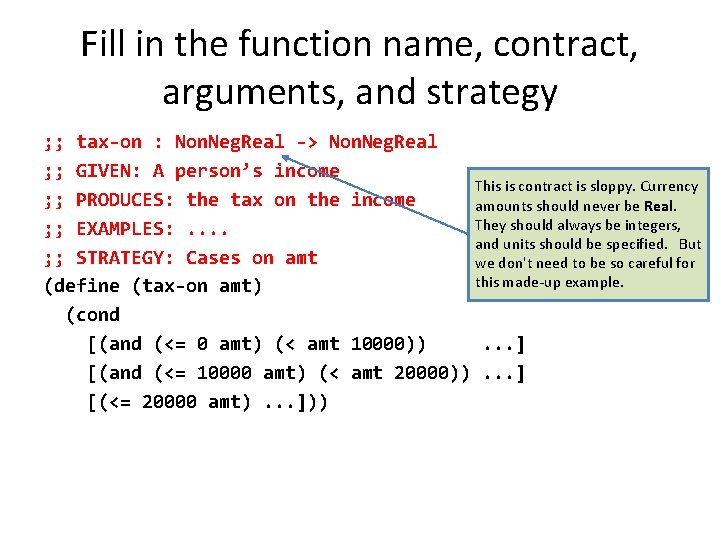Fill in the function name, contract, arguments, and strategy ; ; tax-on : Non. Neg. Real -> Non. Neg. Real ; ; GIVEN: A person’s income This is contract is sloppy. Currency ; ; PRODUCES: the tax on the income amounts should never be Real. They should always be integers, ; ; EXAMPLES: . . and units should be specified. But ; ; STRATEGY: Cases on amt we don't need to be so careful for this made-up example. (define (tax-on amt) (cond [(and (<= 0 amt) (< amt 10000)) . . . ] [(and (<= 10000 amt) (< amt 20000)). . . ] [(<= 20000 amt). . . ]))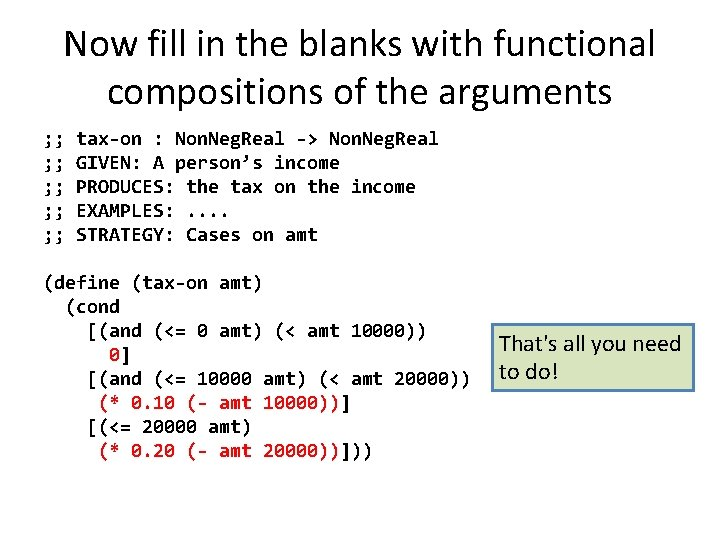Now fill in the blanks with functional compositions of the arguments ; ; tax-on : Non. Neg. Real -> Non. Neg. Real ; ; GIVEN: A person’s income ; ; PRODUCES: the tax on the income ; ; EXAMPLES: . . ; ; STRATEGY: Cases on amt (define (tax-on amt) (cond [(and (<= 0 amt) (< amt 10000)) 0] [(and (<= 10000 amt) (< amt 20000)) (* 0. 10 (- amt 10000))] [(<= 20000 amt) (* 0. 20 (- amt 20000))])) That's all you need to do!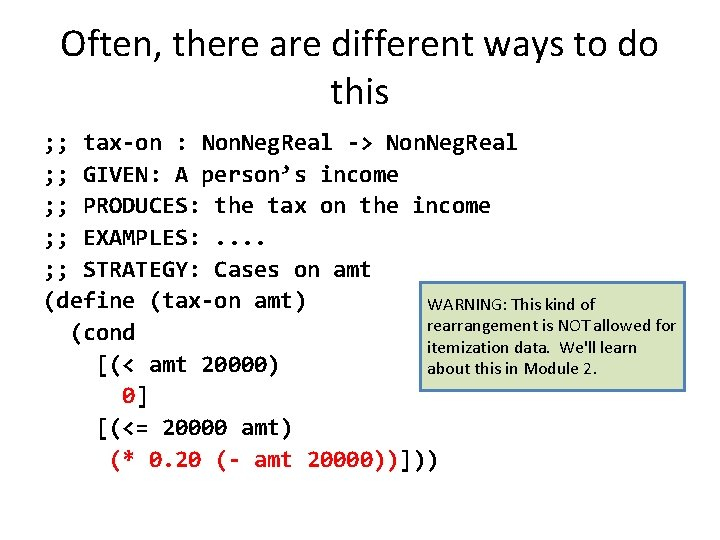Often, there are different ways to do this ; ; tax-on : Non. Neg. Real -> Non. Neg. Real ; ; GIVEN: A person’s income ; ; PRODUCES: the tax on the income ; ; EXAMPLES: . . ; ; STRATEGY: Cases on amt (define (tax-on amt) WARNING: This kind of rearrangement is NOT allowed for (cond itemization data. We'll learn [(< amt 20000) about this in Module 2. 0] [(<= 20000 amt) (* 0. 20 (- amt 20000))]))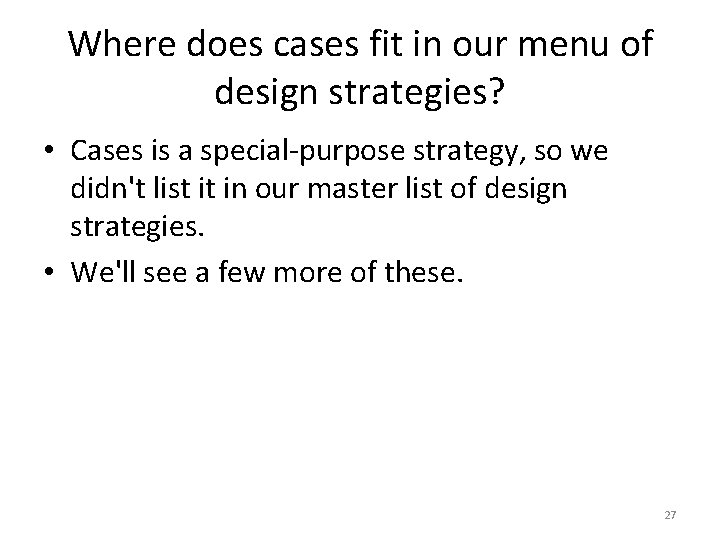Where does cases fit in our menu of design strategies? • Cases is a special-purpose strategy, so we didn't list it in our master list of design strategies. • We'll see a few more of these. 27Summary • In this lesson, you’ve learned – How to use Function Composition to write a function definition. – When a function definition needs to be simplified by using help functions. – How to use Cases to partition a scalar data type. 28